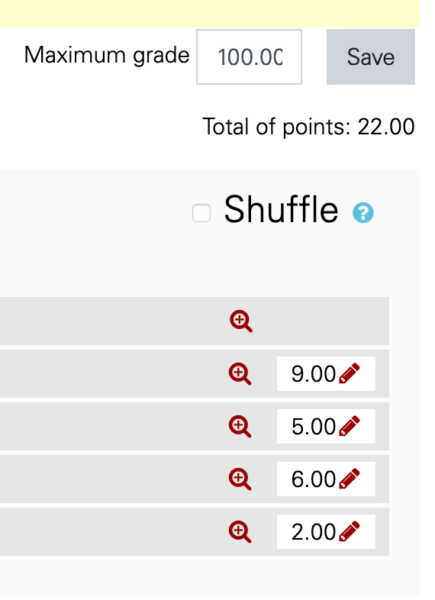# Adjusting point totals on a Moodle quiz

On Moodle quizzes, you can adjust how much each question is worth as well as declare the overall score:• On this quiz, there are 4 questions worth 9, 5, 6, and 2 points respectively.
• Moodle automatically adds these up to say that they are worth a total of 22 points (near the top).
• By clicking the pencil, you can change how much each is worth.
• The maximum grade is how the grade is stored in the grade book. So a student who earns 20 out of 22 points gets a grade of 90.9% -- Moodle does this calculation for you!
• If you want all quizzes to be equally weighted in your gradebook calculations, simply declare them to all have the same maximum grade. Then you don't have to manually force the point distributions on each quiz to be the same.